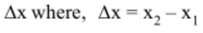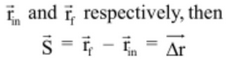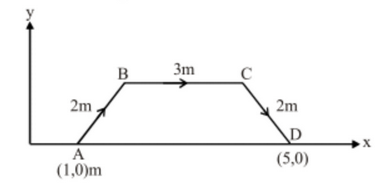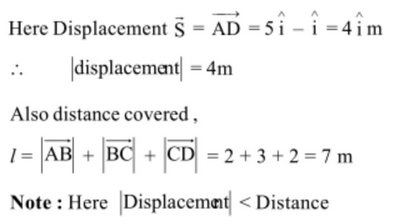# Displacement Vs Distance

Displacement

Displacement is the change in position vector.

Its magnitude is minimum distance between final and initial point, and is directed from initial position to final position.

For a particle moving along x-axis, motion from one position x1 to another position x2 is displacement,If particles move from x1 = 4m to x2 = 12 m, the X = 12m – 4m = +8m. The positive result indicates that the motion is in the positive direction. If the particle then returns to x = 4m, the displacement for the full trip is zero. The actual numbers of meters covered for the full trip is irrelevant displacement involves only the original and final position.

In general, if initial position vector and final position vector areDistance

Distance is the length of path traversed by a body.

It is dependent on path chosen for motion between two fixed points A and B we can have many different values of distance traversed. It is a scalar quantity, as length of path has no indication of direction in it. Its SI unit is meter (m) and dimensions is L.

Eg. Suppose a particle moves from position A to B to C to D.Magnitude of displacement would be equal to distance travelled if there is no change in direction during the whole motion.

In general, |Displacement| <= Distance

Post By : Divya SIngh 30 Jan, 2020 2500 views Physics